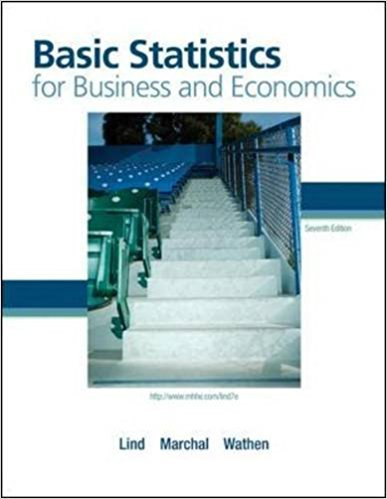×
Get Full Access to Basic Statistics For Business And Economics - 7 Edition - Chapter 3 - Problem 1e
Get Full Access to Basic Statistics For Business And Economics - 7 Edition - Chapter 3 - Problem 1e

×

# Compute the mean of the following population values: 6, 3,ISBN: 9780077384470 64

## Solution for problem 1E Chapter 3

Basic Statistics for Business and Economics | 7th Edition

• Textbook Solutions
• 2901 Step-by-step solutions solved by professors and subject experts
• Get 24/7 help from StudySoup virtual teaching assistantsBasic Statistics for Business and Economics | 7th Edition

4 5 1 391 Reviews
19
5
Problem 1E

Problem 1E

Compute the mean of the following population values: 6, 3, 5, 7, 6.

Step-by-Step Solution:

Problem 1E

Compute the mean of the following population values: 6, 3, 5, 7, 6.

Step by step solution

Step 1 of 3

The given data X is: 6, 3, 5, 7, 6.

The number of observations N are 5.

Step 2 of 3

Step 3 of 3

##### ISBN: 9780077384470

This textbook survival guide was created for the textbook: Basic Statistics for Business and Economics , edition: 7. This full solution covers the following key subjects: compute, mean, population, values. This expansive textbook survival guide covers 6 chapters, and 325 solutions. Basic Statistics for Business and Economics was written by and is associated to the ISBN: 9780077384470. The answer to “Compute the mean of the following population values: 6, 3, 5, 7, 6.” is broken down into a number of easy to follow steps, and 13 words. The full step-by-step solution to problem: 1E from chapter: 3 was answered by , our top Business solution expert on 08/23/17, 08:36AM. Since the solution to 1E from 3 chapter was answered, more than 594 students have viewed the full step-by-step answer.

Unlock Textbook Solution